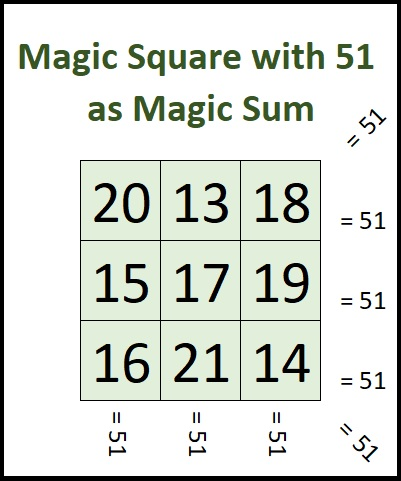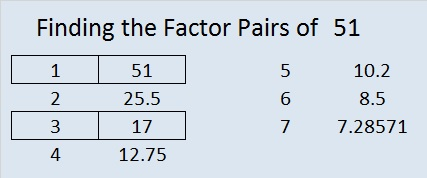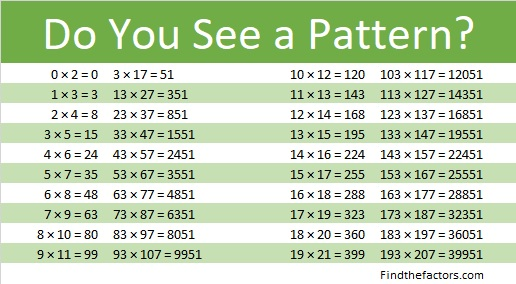# 51 and Magic forcing grid – Maths Magic

Mathematics is full of magic, sometimes more than we even expected. There appears to be a connection between this math trick and 4 x 4 magic squares. I will be interested to see if anyone can form a 4 x 4 magic square from the information provided by The Science Magician in this post. There are 880 ways to make a 4 x 4 magic square, so it seems like it would still be a lot of work. This same process works on a 3 x 3 square and results in numbers that add up to 15 in every case when the numbers from 1 to 9 are used.

If you reverse the digits in 15, you get 51. Can you make a magic square in which the magic sum is 51? Yes, you can! If you use the numbers from 13 to 21, the magic sum will be 51. One such configuration is shown here:Notice that 17 is the number in the middle square and 17 × 3 = 51.

Only multiples of 3 that are greater than or equal to 15 can be magic sums of a 3 × 3 magic square.

51 is a composite number. 51 = 1 x 51 or 3 x 17. Factors of 51: 1, 3, 17, 51. Prime factorization: 51 = 3 x 17.51 is never a clue in the FIND THE FACTORS puzzles.

51 is in this curious pattern:This site uses Akismet to reduce spam. Learn how your comment data is processed.